# Gauge transformation

(diff) ← Older revision | Latest revision (diff) | Newer revision → (diff)

A transformation in classical and quantum field theory which alters non-observable properties of fields (e.g. potentials) without changing the physically-meaningful measurable magnitudes (e.g. intensities). The name "gauge transformation" (or "gradient transformation" ) arose in the classical theory of electromagnetic fields. In this theory the four-dimensional electromagnetic vector potential,, is introduced in an ambiguous manner, since the so-called gauge transformations of the second kind:(1)

with an arbitrary functionhaving partial derivatives of the first and second orders, do not affect the values of the components of the anti-symmetric electromagnetic field tensor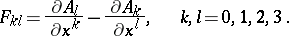These components are equal to the physically-observable components of the intensity vectors of the electric field,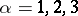, and of the magnetic field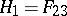;;. Under a gauge transformation of the second kind the field equations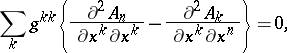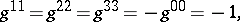remain unchanged; this is the so-called gauge invariance of field theory. If the functionis suitably chosen, the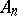can be made to satisfy some additional condition (the so-called gauge condition), whereby the form of the field equations can be simplified. Thus, the Lorentz condition, which is linear with respect to the vector potential,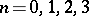, stating that its four-dimensional divergence vanishes:(2)

leads to d'Alembert's equation for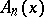(cf. d'Alembert operator):(3)

The Lorentz condition does not completely determine thesince, in theory, the invariance with respect to a so-called specialized gauge transformation (1) of the second kind with a function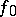that satisfies d'Alembert's equationremains. However, ifis selected in a certain manner (for a Lorentz coordinate system), the condition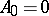will be met. The Lorentz condition (2) is then reduced to the condition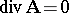for the three-dimensional vector potential, viz. the electromagnetic field is to be transverse. If the field is complex-valued, the theory must also be invariant with respect to gauge transformations of the first kind for the wave functions of the field and their derivatives: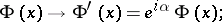(4)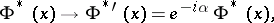since all the observable dynamic magnitudes must (in view of the condition of realness (Hermiticity)) be expressed solely in forms that are bilinear with respect to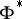and. Owing to the general principles of mechanics, the condition of invariance of a field theory with respect to the gauge transformations of the first kind means that certain observable physical magnitudes must be conserved — such as (electric) charges, which are expressed in terms of field functions, or, in other words, that conservation laws of these charges must exist. The corresponding Lagrangians and field equations should be invariant with respect to gauge transformations. For instance, in the case of complex-valued fields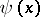interacting with the electromagnetic fieldthat correspond to electric charges, the Lagrangians of the free fields and the Lagrangian of interaction and the field equation should be invariant with respect to gauge transformations of the type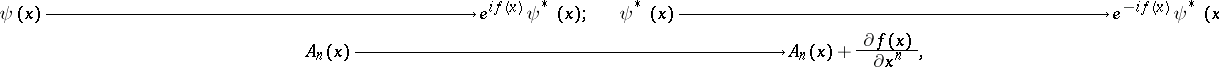(5)

i.e. with respect to the gauge transformations (1) and (4), where the phase multiplier in (4) may depend on the four-dimensional space-time coordinates and should coincide with the arbitrary scalar functions forming part of (1). In such a case the law of conservation of electric charge is fulfilled in the system of fieldsand. The gauge transformations (5) form an Abelian group of transformations in the sense that the gauge function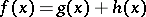describes a gauge transformation representing two gauge transformations with functionsandperformed in any order. In constructing theories of interacting fields which are more involved than the example given above, a necessary condition for meeting the respective conditions of the conservation of any charges is the invariance of the Lagrangians and the field equations with respect to gauge transformations in which the gauge functionsare operators.

How to Cite This Entry:
Gauge transformation. Encyclopedia of Mathematics. URL: http://encyclopediaofmath.org/index.php?title=Gauge_transformation&oldid=12707
This article was adapted from an original article by V.D. Kukin (originator), which appeared in Encyclopedia of Mathematics - ISBN 1402006098. See original article